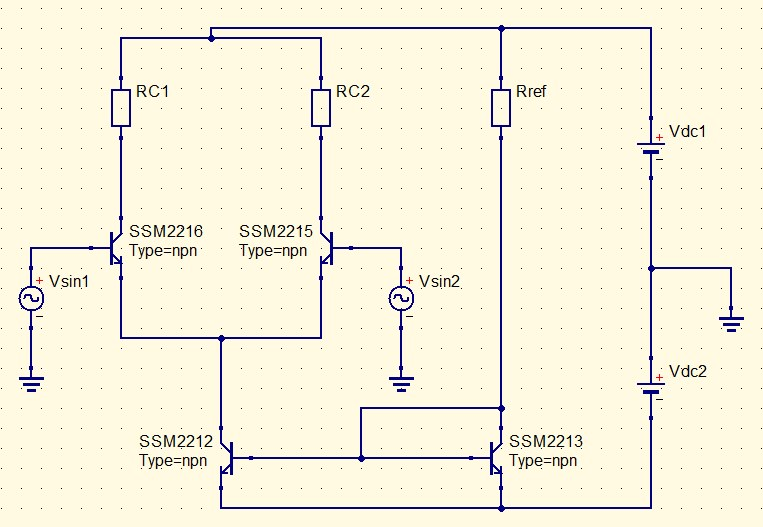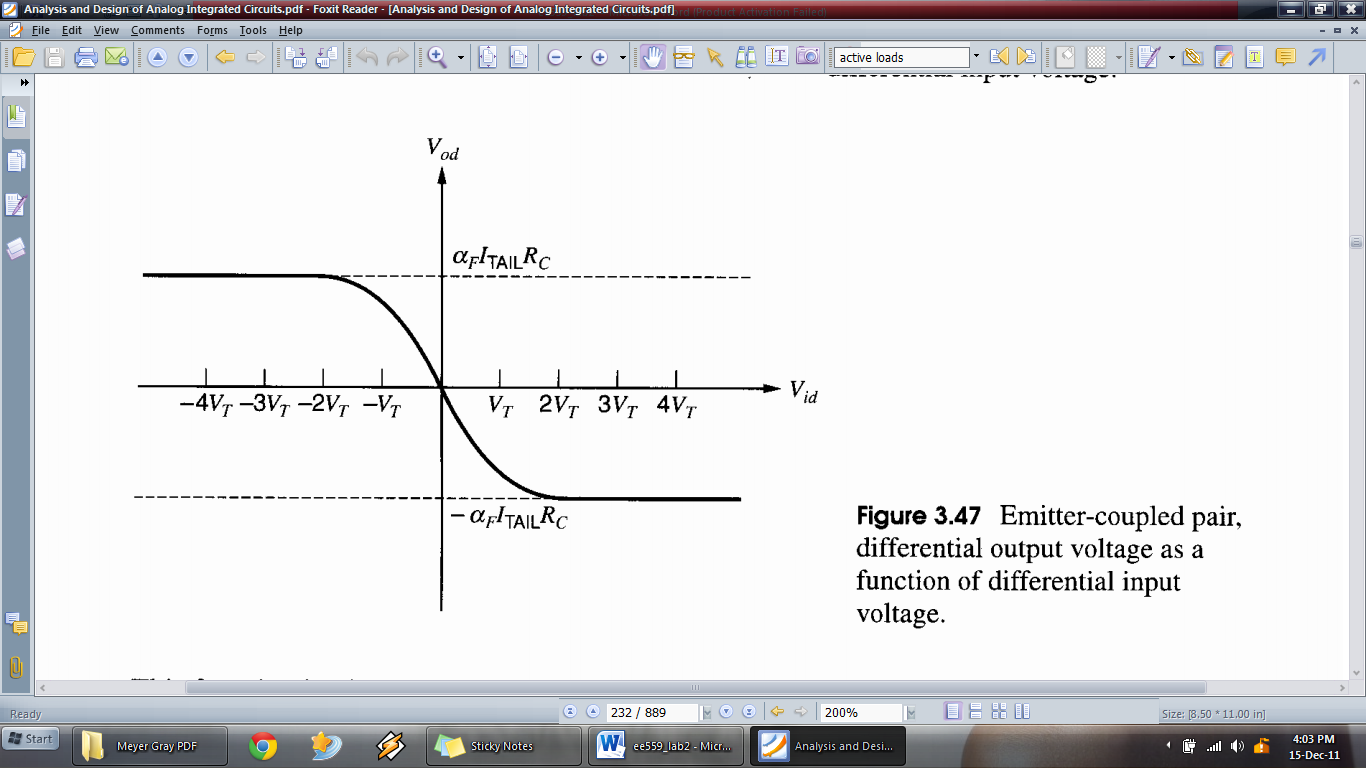EE559 – Integrated Analog Electronic Circuits

Mini Project

Electronics laboratory

OBJECTIVE:

1. To become familiarize with designing a long tail pair for given parameters.
2. To implement the hardware setup for long tail pair.
3. To observe the characteristics of the implemented long tail pair.

-Maximum undistorted output swing.

-DC voltage gain.

-Common mode rejection ratio.

-3dB bandwidth.

APPARATUS:

1. SSM2212 SMD dual transistors (2 ICs)
2. 680 Ohm 1206 SMD resistors
3. 1.8 kOhm 1206 SMD resistors
4. 6.8 kOhm 1206 SMD resistors

CIRCUIT DIAGRAM:Figure1. Schematic diagram of the long tail pair

THEORY:

Using KVL ……….. (1)

Using KCL ……….. (2)

Trans-conductance ……….. (3)

DC Voltage gain ……….. (4)

Maximum Undistorted

Output swing ……….. (5)Figure 2. Relevant Graphs and Equations

PROCEDURE:

1. Select and calculate Vdc1, Vdc2, Rref and Rc values for the given specifications.
• DC voltage gain (AV) : 10 ( 20log(AV) = 20 dB )
• Maximum undistorted output swing : 1V
1. Simulate above circuit using QUCS software and observe following parameters.
• Maximum undistorted output swing.
• DC voltage gain
• Input and output waveforms for 200 Hz
• Differential gain for 0 to 100MHz
• Common mode gain for 0 to 100MHz
• CMRR for 0 to 100MHz
• 3dB bandwidth
1. Use a PCB layout designing software (eg. PROTEUS) to design the PCB layout.

(Note: You should show this design to instructor before implementing hardware)

1. Using selected resistor values and transistor ICs implement the hardware.
2. Take following observations to verify the operation of the long tail pair.
1. Observe output differential voltage by applying -200mV to 200mv input differential DC voltage in the steps of 10mV and plot input differential voltage vs. output differential voltage in order to observe maximum undistorted output voltage swing.
2. From the above graph identify DC voltage gain.
3. Apply 10mV differential DC input voltage and observe the amplification.
4. Apply 200Hz 10mV sinusoidal differential input and observe the output differential waveform.
5. For differential mode change the frequency of the input differential sinusoidal and observe output waveform for 0, 10Hz, 100Hz, 1 kHz, 10 kHz, 100 kHz, 1 MHz, 10 MHz, and 100 MHz and plot frequency (dB) vs. Differential mode gain plot.
6. For common mode change the frequency and observe output waveform for 0, 10Hz, 100Hz, 1 kHz, 10 kHz, 100 kHz, 1 MHz, 10 MHz, and 100 MHz and plot frequency (dB) vs. Common mode gain plot.
7. Using above values obtain the frequency (dB) vs. CMRR plot.
8. Using the results obtain the 3dB bandwidth of the differential amplifier.